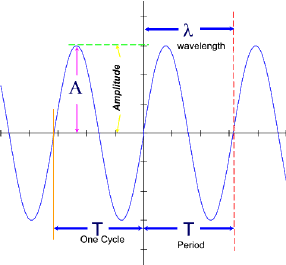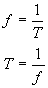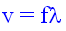#### Waves & Pulses

A wave is a disturbance in a medium that transfers energy.  These "disturbances" can be analyzed as Simple Harmonic Motion.

The smallest part of a wave, acting on one single particle of a medium is called a pulse.  The sum of all pulses make up the wave pattern.

Here is an example of a transverse pulse.  Here you will see the particle being displaced perpendicularly to the direction of travel of the pulse.

There are three main types of waves:

1. Longitudinal
2. Transverse
3. Surface

In longitudinal waves the particles of the medium move perpendicularly to the motion of the wave.

Example: sound is a longitudinal wave

In transverse waves the particles of the medium move parallel to the motion of the wave.

Example: a guitar string vibrating when struck

In surface waves the particles of the medium move in circular patterns relative to the motion of the wave

Example: water waves created by a rock dropped in a pondExternal resources: http://www.acs.psu.edu/drussell/demos/waves/wavemotion.html

#### Period, Frequency, and AmplitudeProperties of a Wave

Refer to the above diagram

Amplitude: The maximum displacement (measured in linear units --meters, centimeters, nanometers, etc.) of the particles of a medium due to the action of a wave or pulse or other harmonic disturbance.

#### In mathematical symbols:#### The Universal Wave Equation:

The universal wave equation tells us that the speed of a wave depends on its frequency and its wavelength.  This equation can be derived from simple equations of motion.

1. Recall that  V = d/t     (total distance/total time).
2. Consider one full cycle of a wave or one wavelength.  The total time elapsed in this cycle is the period of the wave (T) and
3. Recall that   T = 1/f
4. The total distance traveled in one cycle is in fact the wavelength l.

Now, substituting these symbols in the equation  v = d/t  we obtainThe equationis known as the Universal Wave Equation because it applies "universally" to all types of waves traveling in any medium.